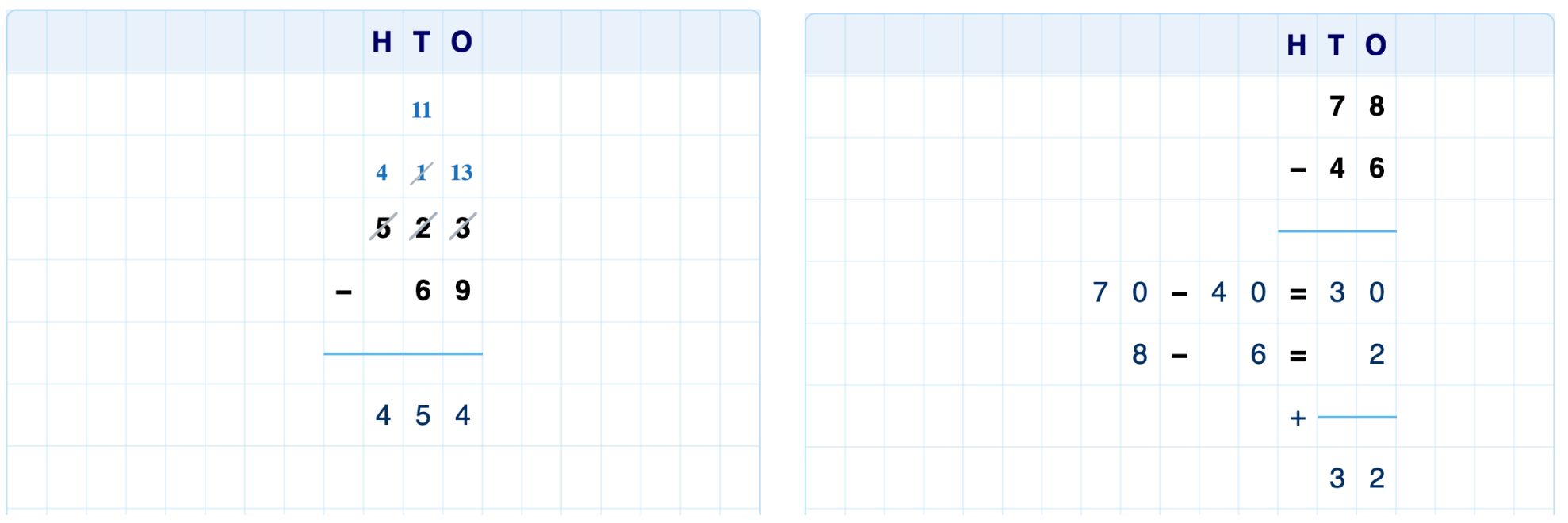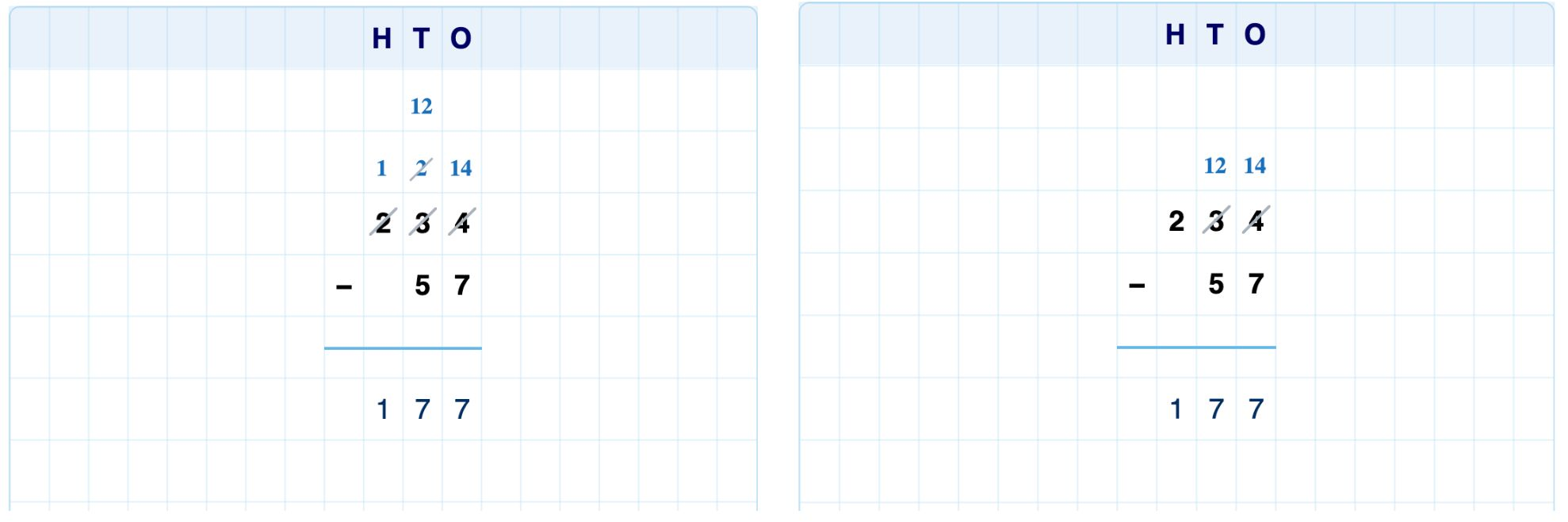# Multi-digit subtraction

## Introduction

Multi-digit subtraction shares many of the features of multi-digit addition, such as:

• Short and extended forms
• Options to specify elements as optional or required
• Options to specify what part of the problem is visible at the start
• Option to display a place value ribbon.Figure: Short and extended forms for subtraction.

## Regrouping

The short form of subtraction uses regrouping, also known as borrowing, when the digit being subtracted is larger than the digit above it. When students type in the regrouping cells, the digits below them are automatically scratched through.

You can configure if students must show regrouping in their solution or if this is optional.Figure: A solution with regrouping required (left) and optional (right).